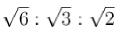Courses

# Crystals MCQ Level – 2

## 10 Questions MCQ Test Solid State Physics, Devices & Electronics | Crystals MCQ Level – 2

Description
This mock test of Crystals MCQ Level – 2 for IIT JAM helps you for every IIT JAM entrance exam. This contains 10 Multiple Choice Questions for IIT JAM Crystals MCQ Level – 2 (mcq) to study with solutions a complete question bank. The solved questions answers in this Crystals MCQ Level – 2 quiz give you a good mix of easy questions and tough questions. IIT JAM students definitely take this Crystals MCQ Level – 2 exercise for a better result in the exam. You can find other Crystals MCQ Level – 2 extra questions, long questions & short questions for IIT JAM on EduRev as well by searching above.
QUESTION: 1

### What is the second nearest neighbour distance in bcc crystal whose conventional unit cell parameter is a?

Solution:

In a bcc structure, for any atom at the corner of the lattice, nearest neighbour is at the centre of the lattice which is at a distance of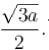The second nearest neighbour is at the adjacent corners of the lattice at a distance of 'a'.

QUESTION: 2

### The number of atoms per unit area of the plane [0 1 0] of a simple cubic crystal is :

Solution:

[0 1 0] is the miller indices of the plane, hence, the intercept will be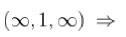the plane is in x-z plane passing through y = 1.
Now number of atoms in the plane for simple cubic lattice is =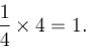⇒ Number of atoms per unit area of plane [0 1 0] of simple cubic lattice is,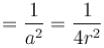(since, a = 2r).
The correct answer is: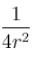QUESTION: 3

### If a two - dimensional lattice has the basic vector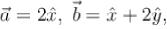hen the vectors of the reciprocal lattice are respectively;

Solution: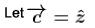The vectors of reciprocal lattice are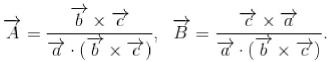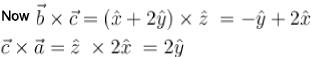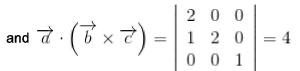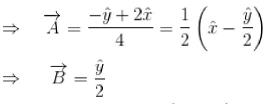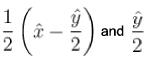QUESTION: 4

In crystallographic notations, the vector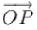is the cubic cell shown is the figure is :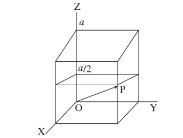Solution:

Coordinates of point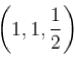is , hence, in crystal notation the plane is [2 2 1]
The correct answer is: [2 2 1]

QUESTION: 5

[3 2 6] are the miller indices of a plane, the intercept mode by the plane on the 3 crystallographic axes are:

Solution:

Miller indices are (3, 2, 6)
Hence, crystallographic axis are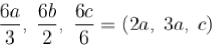The correct answer is: (2a, 3b, c)

QUESTION: 6

Consider the atomic packing factor (APF) of the following crystal structures :
P. Simple cubic
Q. Body centred cubic
R. Face centred cubic
S. Diamond T. Hexagonal close packed
Q. Which two of the above structure have equal APF?

Solution:

simple cubic → 52%, bcc → 68%, fcc → 74%, diamond → 34%, hcp 74%
The correct answer is: R and T

QUESTION: 7

If d be the space between parallel lattice planes and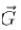be reciprocal lattice vector, then which of the following relations are true?

Solution:

If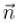is unit vector normal to the plane, then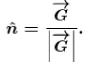If d is the interplanar. distance,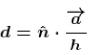where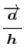is the translation vector along one of the unit axis.
Here the planes are perpendicular to this unit axis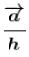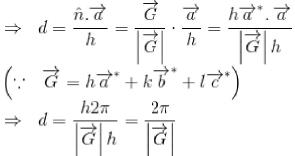The correct answer is: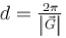QUESTION: 8

Volume of primitive cell of bcc structure having conventional lattice parameter a is :

Solution:

For bcc structure :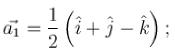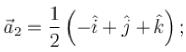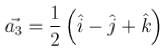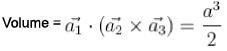The correct answer is: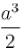QUESTION: 9

For a NaCI crystal, the cell edge a = 0.563 nm. The smallest angle at which Bragg reflection can occur corresponds to a set of planes whose indices are :

Solution: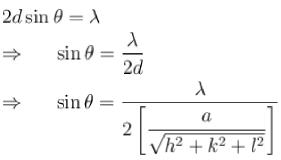For θ to be small,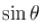is also small hence,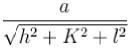is minimum and this minimum of
h =1, Ac = I = 0
The correct answer is: [1 0 0]

QUESTION: 10

For a simple cubic lattice d100 : d110 : d111 is.

Solution: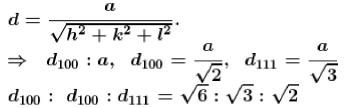The correct answer is: